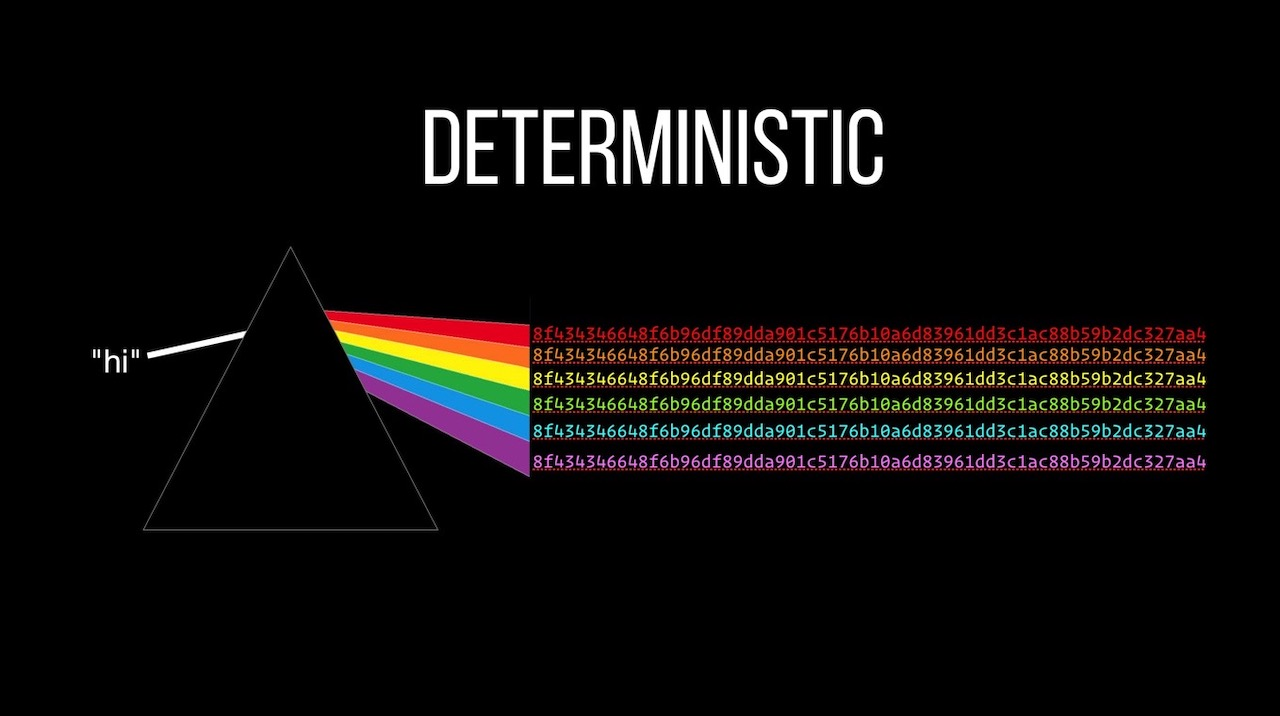👋🏼

# Hashing and Asymmetric Encryption

I've used hashes for a long time and had a general understanding of what they do... but that's about it. I knew I needed to hash passwords (and other secure data) if I was going to store them in my database... but that's about all.

I was never sure which algorithms to use nor why... so I dug in and found out. That's the first part of this video.

The second is asymmetric encryption: encoding with a public key and decoding with a second, private key. Absolutely ground-breaking idea! The math is amazingly straightforward too... although I will admit to struggling to explain some of it.

## The Code

To produce a simple hash using Node:

``````const crypto = require("crypto");

const hash = crypto.createHash("sha256")
.update("hi")
.digest("hex");

console.log(hash);
``````

Hashing a password with PBKDF2 using a million rounds to slow things down:

``````const crypto = require("crypto");

return new Promise(function(resolve, reject){
crypto.pbkdf2(pw,"super-secret-salt",1000000,32,"sha512", function(err, buffer){
if(err) reject(err);
else resolve(buffer.toString("hex"))
});
})
}

``````

Hashing using scrypt, assigning a cost:

``````const hashPassword = function(pw){
return new Promise(function(resolve, reject){
crypto.scrypt(pw,"super-secret-salt",32, {cost: 2**14}, function(err, buffer){
if(err) reject(err);
else resolve(buffer.toString("hex"))
});
})
}
``````

Creating a checksum using MD5:

``````const crypto = require("crypto");
const fs = require("fs");

const checksum = function(data){
return crypto.createHash("md5").update(data, "utf8").digest("hex")
}

console.log(checksum(data));
``````

A super-simple mining operation (meant for demonstration only) for blockchain stuff:

``````const crypto = require("crypto");

const createHash = (block) => {
//need to pass a string to our
const hashValue = JSON.stringify(block)
return crypto.createHash("sha256")
.update(hashValue)
.digest("base64");
}

const mine = (block, difficulty = 2) => {
let found = false, start = new Date().getTime();
//we're looking for a string of 0s so let's create the pattern
//I could use Regex but I'm not that good
console.log("Looking for a hash starting with", lookingFor);
const duration = new Date().getTime() - start;

while(!found){
const possibleHash = createHash(block);
found = possibleHash.substring(0, difficulty) === lookingFor;
if(found){
block.hashKey = possibleHash;
return block;
}
block.nonce += 1;
//10 second kill switch
if(duration > 10000) return "Didn't find it under 10s"
}
}

const block = {
transactions: [
{from: "me", to: "you", amount: 10.00},
{from: "you", to: "me", amount: 5.00}
],
timestamp: 1609702546153, //if this changes the hash changes
nonce: 0,
previousKey: "00RSDThMVcQAvoocD3klO/6pjJ4a8pRbZ3ykk3XXhXE="
}
//let's see how long this takes
const start = new Date().getTime();
//let's do it!
const result = mine(block, 4);
const duration = new Date().getTime() - start;
console.log(`That took duration \${duration}ms`);
console.log(result);
``````

And finally, our trip through the basics of RSA using super small primes:

``````//resources
//https://www.cs.drexel.edu/~jpopyack/IntroCS/HW/RSAWorksheet.html
//https://www.cryptool.org/en/cto/highlights/rsa-step-by-step
//let's find two relatively prime numbers, e and d, such that
//e % d mod r === 1
//this is the cornerstone of RSA
var findEandD = function(r) {
//These are common candidates for e, which can be autoset
//and typically 65537 is used, but we'll
//start small for speed
const possibleEs = [3n,5n,17n,257n,65537n]
//now, loop over the possible e's so we can find our d
for(let e of possibleEs){
//we want to find a coprime for e, so let's factor it
//up to r and see if e % r is 1
//if it is, we found our coprime
for (let d = 1n; d < r; d++) {
const candidate = e * d;
if (candidate % r == 1n) return {e: e, d: d};
}
}
assert.fail("We shouldn't reach this point")
}

const p = 499n;
const q = 491n;
assert.notStrictEqual(p,q, "p and q must be different primes");

//our public key, N
const N = p * q;

//Euler's totient for deriving r
//r = phi(n) = (p-1) * (q-1)
const r = (p-1n) * (q-1n);

//now we can calculate e and d for our private key
const {e,d} = findEandD(r);

//our message
const M = 25n;

console.log("e",e);
console.log("d",d);
console.log("N",N);
console.log("r",r);

console.log("M", M);

//the RSA algorithm
const encrypted = M**e % N;
const decrypted = encrypted**d % N;

console.log("Encrypted",encrypted);
console.log("Decrypted",decrypted);
``````

I cite a number of articles in this video - hard not to. But here they are if you want to read more: